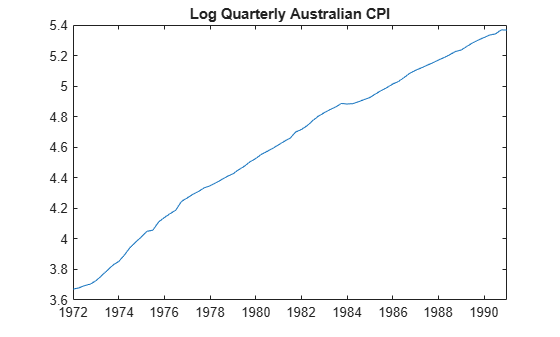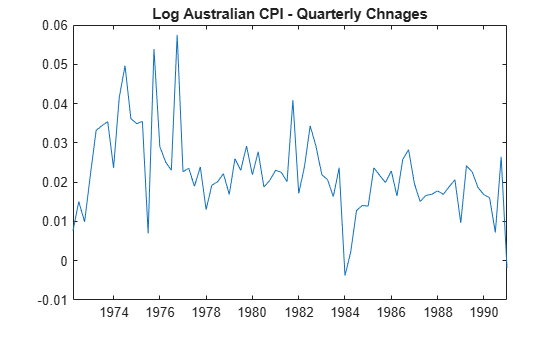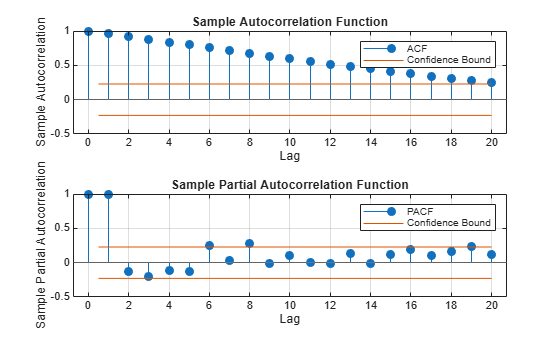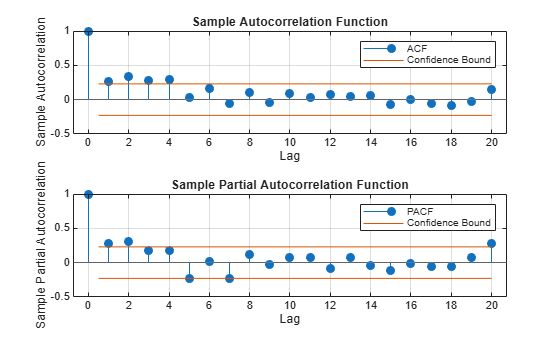# Select ARIMA Model for Time Series Using Box-Jenkins Methodology

This example shows how to use the Box-Jenkins methodology to select an ARIMA model. The time series is the log quarterly Australian Consumer Price Index (CPI) measured from 1972 and 1991.

### Box-Jenkins Methodology

The Box-Jenkins methodology  is a five-step process for identifying, selecting, and assessing conditional mean models (for discrete, univariate time series data).

1. Determine whether the time series is stationary. If the series is not stationary, successively difference it to attain stationarity. The sample autocorrelation function (ACF) and partial autocorrelation function (PACF) of a stationary series decay exponentially (or cut off completely after a few lags).

2. Identify a stationary conditional mean model for the series. The sample ACF and PACF functions can help with this selection. For an autoregressive (AR) process, the sample ACF decays gradually, but the sample PACF cuts off after a few lags. Conversely, for a moving average (MA) process, the sample ACF cuts off after a few lags, but the sample PACF decays gradually. If both the ACF and PACF decay gradually, consider an ARMA model.

3. Create a model template for estimation, and then fit the model to the series. When fitting nonstationary models in Econometrics Toolbox™, you do not need to manually difference the series and fit a stationary model. Instead, you can use the series on the original scale, and create an `arima` model object with the desired degree of nonseasonal and seasonal differencing. Fitting an ARIMA model directly is advantageous for forecasting: forecasts are returned on the original scale (not differenced).

4. Conduct goodness-of-fit checks to ensure the model describes the series adequately. Residuals should be uncorrelated, homoscedastic, and normally distributed with constant mean and variance. If the residuals are not normally distributed, you can change the innovation distribution to a Student’s $\mathit{t}$.

5. After choosing a model—and checking its fit and forecasting ability—you can use the model to forecast or generate Monte Carlo simulations over a future time horizon.

Load and plot the Australian CPI data.

```load Data_JAustralian y = DataTable.PAU; T = length(y); figure plot(y) h1 = gca; h1.XLim = [0,T]; h1.XTick = 1:10:T; h1.XTickLabel = datestr(dates(1:10:T),17); title('Log Quarterly Australian CPI')```The series is nonstationary, with a clear upward trend.

### Plot the Sample ACF and PACF

Plot the sample autocorrelation function (ACF) and partial autocorrelation function (PACF) for the CPI series.

```figure subplot(2,1,1) autocorr(y) subplot(2,1,2) parcorr(y)```The significant, linearly decaying sample ACF indicates a nonstationary process.

### Difference the Data

Take a first difference of the data, and plot the differenced series.

```dY = diff(y); figure plot(dY) h2 = gca; h2.XLim = [0,T]; h2.XTick = 1:10:T; h2.XTickLabel = datestr(dates(2:10:T),17); title('Differenced Log Quarterly Australian CPI')```Differencing removes the linear trend. The differenced series appears more stationary.

### Plot the Sample ACF and PACF of the Differenced Series

Plot the sample ACF and PACF of the differenced series to look for behavior more consistent with a stationary process.

```figure subplot(2,1,1) autocorr(dY) subplot(2,1,2) parcorr(dY)```The sample ACF of the differenced series decays more quickly. The sample PACF cuts off after lag 2. This behavior is consistent with a second-degree autoregressive (AR(2)) model.

### Specify and Estimate an ARIMA(2,1,0) Model

Specify, and then estimate, an ARIMA(2,1,0) model for the log quarterly Australian CPI. This model has one degree of nonseasonal differencing and two AR lags. By default, the innovation distribution is Gaussian with a constant variance.

```Mdl = arima(2,1,0); EstMdl = estimate(Mdl,y);```
``` ARIMA(2,1,0) Model (Gaussian Distribution): Value StandardError TStatistic PValue __________ _____________ __________ __________ Constant 0.010072 0.0032802 3.0707 0.0021356 AR{1} 0.21206 0.095428 2.2222 0.02627 AR{2} 0.33728 0.10378 3.2499 0.0011543 Variance 9.2302e-05 1.1112e-05 8.3066 9.8491e-17 ```

Both AR coefficients are significant at the 0.05 significance level.

### Check Goodness of Fit

Infer the residuals from the fitted model. Check that the residuals are normally distributed and uncorrelated.

```res = infer(EstMdl,y); figure subplot(2,2,1) plot(res./sqrt(EstMdl.Variance)) title('Standardized Residuals') subplot(2,2,2) qqplot(res) subplot(2,2,3) autocorr(res) subplot(2,2,4) parcorr(res) hvec = findall(gcf,'Type','axes'); set(hvec,'TitleFontSizeMultiplier',0.8,... 'LabelFontSizeMultiplier',0.8);```The residuals are reasonably normally distributed and uncorrelated.

### Generate Forecasts

Generate forecasts and approximate 95% forecast intervals for the next 4 years (16 quarters).

```[yF,yMSE] = forecast(EstMdl,16,y); UB = yF + 1.96*sqrt(yMSE); LB = yF - 1.96*sqrt(yMSE); figure h4 = plot(y,'Color',[.75,.75,.75]); hold on h5 = plot(78:93,yF,'r','LineWidth',2); h6 = plot(78:93,UB,'k--','LineWidth',1.5); plot(78:93,LB,'k--','LineWidth',1.5); fDates = [dates; dates(T) + cumsum(diff(dates(T-16:T)))]; h7 = gca; h7.XTick = 1:10:(T+16); h7.XTickLabel = datestr(fDates(1:10:end),17); legend([h4,h5,h6],'Log CPI','Forecast',... 'Forecast Interval','Location','Northwest') title('Log Australian CPI Forecast') hold off```Box, George E. P., Gwilym M. Jenkins, and Gregory C. Reinsel. Time Series Analysis: Forecasting and Control. 3rd ed. Englewood Cliffs, NJ: Prentice Hall, 1994.